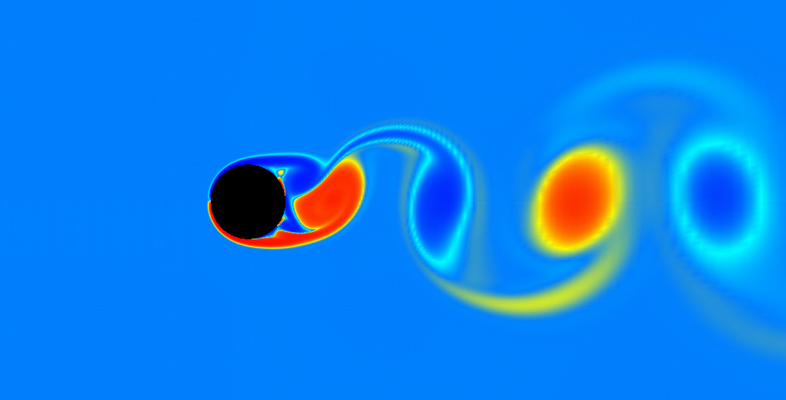### Become an OU studentKinematics of fluids

Start this free course now. Just create an account and sign in. Enrol on the course to track your learning.

# Conclusion

Successful study of this free OpenLearn course, Kinematics of fluids, should enhance your skills in communicating mathematical ideas clearly and succinctly, expressing problems in mathematical language and interpreting mathematical results in real-world terms.

You should now be able to:

• derive the pathlines and the streamlines in Cartesian and polar form from a velocity field
• derive the stream function from a velocity field
• model different flows from a combination of uniform flows, sources, sinks and doublets
• use the continuity equation to determine whether an inviscid flow is incompressible
• understand how Euler’s equation is derived, what it represents, and use it to find the pressure distribution from a velocity.

This free OpenLearn course is an extract from the Open University course MST326 Mathematical methods and fluid mechanics [Tip: hold Ctrl and click a link to open it in a new tab. (Hide tip)] .

If you feel you are ready to move on in your study of applied mathematics but don’t have time to study a full Open University course at this time, you might like to study the free OpenLearn courses Linear programming – the basic ideas and Introduction to the calculus of variations.

If you are interested in pure mathematics, you might like to try the free OpenLearn courses Number theory, Group theory, Rings and polynomials and Metric spaces and continuity.

If you are interested in statistics, you might like to study the free OpenLearn courses Modelling events in time, Univariate continuous distribution theory and Point estimation.